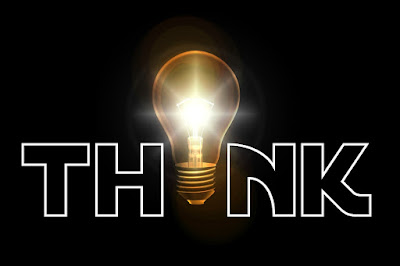## Wednesday, 16 May 2018

### Material and Components Multiple Choice Questions

Electronics and Communication Engineering Questions and Answers for Examination.

1. A capacitor that stores 0.5 C at 10 Volt has a capacitance of _____ farad?

a. 5
b. 20
c. 10
d. 0.05

2. The main function of a capacitor is to _____.

a. Block current flow
b. Help current flow
c. Store energy
d. Dissipate heat

3. The reactance of a coil at 100 Hz is 20 Ohm. Its reactance at 1 Khz would be _____ Ohm.

a. 2
b. 10
c. 40
d. 200

4. A coil of 2 H develops a counter of 4V in it. The rate of change of current through it must be _____ A/s.

a. 2
b. 0.5
c. 8
d. 1

5. A coil has an Inductance of  1 H if current changing at the rating of 2 A/s induces.

a. 1 V
b. 2 V in another coil
c. 2 V
d. 1/2 V

6.  An Inducatnce of a coil depends. Point out the incorrect answer.

a. Directly on the square of the number of its turn
b. Directly on the cross-sectional area of the core
c. Directly on the thickness of the wire used
d. Inversely as the length of the core

7. Ferrite cores commonly used in high frequencies

a. increase core losses
b. decrease core losses
c. decrease inductance
d. increase resistance

8. While measuring resistance with an ohm meter one should not touch test leads because_____.

a. of the possibility of electric shock
b. it may lower the ohm meter rating
c. it may ground the resistor
d. it may short the resistor being checked

9. When checked with an ohm meter, an open resistor leads.

a. infinite
b. zero
c. low but not zero
d. high but not infinite

10. If the current through a resistor is halved, wattage developed by it would be

a. half
c. cut to one-fourth
d. doubled

1. 3
2. 3
3. 2
4. 4
5. 1
6. 2
7. 2
8. 3
9. 3
10. 4